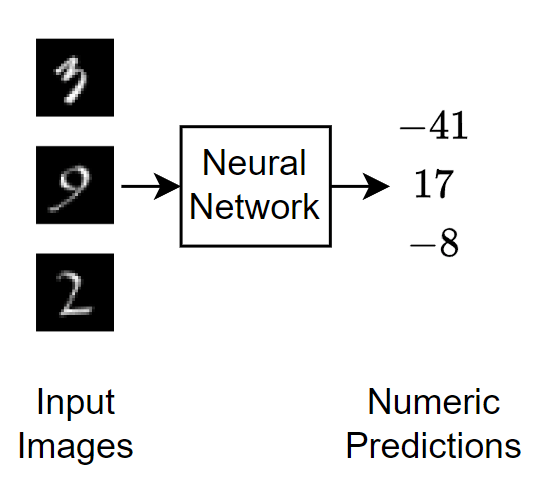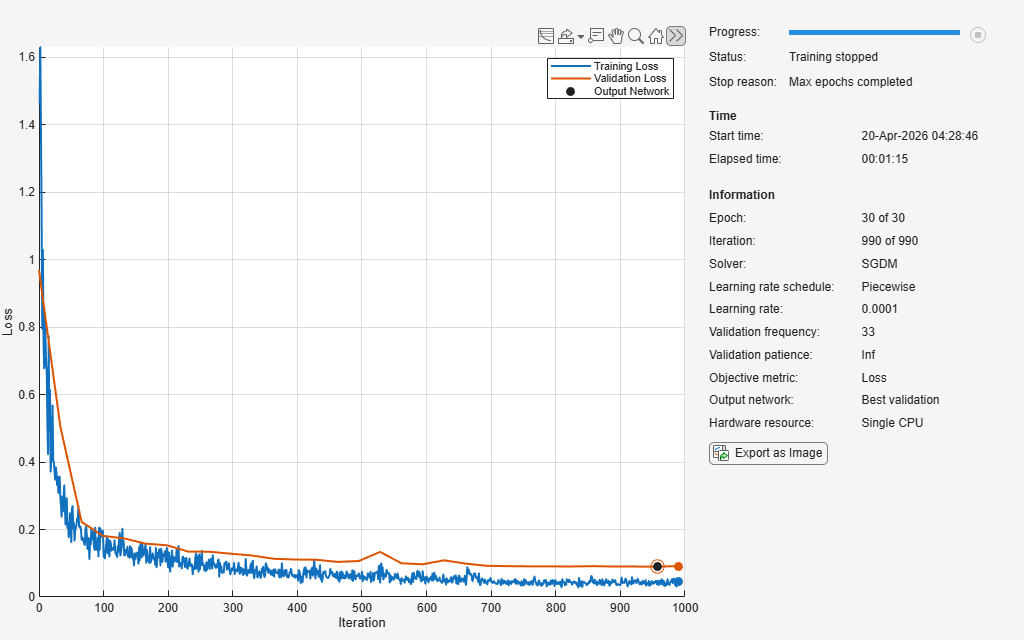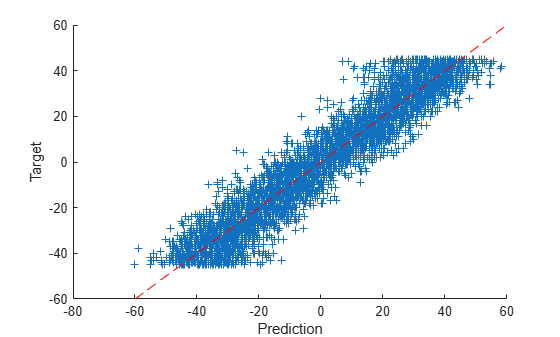# Train Convolutional Neural Network for Regression

This example shows how to fit a regression model using convolutional neural networks to predict the angles of rotation of handwritten digits.

Convolutional neural networks (CNNs, or ConvNets) are essential tools for deep learning, and are especially suited for analyzing image data. For example, you can use CNNs to classify images. To predict continuous data, such as angles and distances, you can include a regression layer at the end of the network.

The example constructs a convolutional neural network architecture, trains a network, and uses the trained network to predict angles of rotated handwritten digits. These predictions are useful for optical character recognition.

Optionally, you can use `imrotate` (Image Processing Toolbox™) to rotate the images, and `boxplot` (Statistics and Machine Learning Toolbox™) to create a residual box plot.

The data set contains synthetic images of handwritten digits together with the corresponding angles (in degrees) by which each image is rotated.

Load the training and validation images as 4-D arrays using `digitTrain4DArrayData` and `digitTest4DArrayData`. The outputs `YTrain `and `YValidation` are the rotation angles in degrees. The training and validation data sets each contain 5000 images.

```[XTrain,~,YTrain] = digitTrain4DArrayData; [XValidation,~,YValidation] = digitTest4DArrayData;```

Display 20 random training images using `imshow`.

```numTrainImages = numel(YTrain); figure idx = randperm(numTrainImages,20); for i = 1:numel(idx) subplot(4,5,i) imshow(XTrain(:,:,:,idx(i))) end```### Check Data Normalization

When training neural networks, it often helps to make sure that your data is normalized in all stages of the network. Normalization helps stabilize and speed up network training using gradient descent. If your data is poorly scaled, then the loss can become `NaN` and the network parameters can diverge during training. Common ways of normalizing data include rescaling the data so that its range becomes [0,1] or so that it has a mean of zero and standard deviation of one. You can normalize the following data:

• Input data. Normalize the predictors before you input them to the network. In this example, the input images are already normalized to the range [0,1].

• Layer outputs. You can normalize the outputs of each convolutional and fully connected layer by using a batch normalization layer.

• Responses. If you use batch normalization layers to normalize the layer outputs in the end of the network, then the predictions of the network are normalized when training starts. If the response has a very different scale from these predictions, then network training can fail to converge. If your response is poorly scaled, then try normalizing it and see if network training improves. If you normalize the response before training, then you must transform the predictions of the trained network to obtain the predictions of the original response.

Plot the distribution of the response. The response (the rotation angle in degrees) is approximately uniformly distributed between -45 and 45, which works well without needing normalization. In classification problems, the outputs are class probabilities, which are always normalized.

```figure histogram(YTrain) axis tight ylabel('Counts') xlabel('Rotation Angle')```In general, the data does not have to be exactly normalized. However, if you train the network in this example to predict `100*YTrain` or `YTrain+500` instead of `YTrain`, then the loss becomes `NaN` and the network parameters diverge when training starts. These results occur even though the only difference between a network predicting aY + b and a network predicting Y is a simple rescaling of the weights and biases of the final fully connected layer.

If the distribution of the input or response is very uneven or skewed, you can also perform nonlinear transformations (for example, taking logarithms) to the data before training the network.

### Create Network Layers

To solve the regression problem, create the layers of the network and include a regression layer at the end of the network.

The first layer defines the size and type of the input data. The input images are 28-by-28-by-1. Create an image input layer of the same size as the training images.

The middle layers of the network define the core architecture of the network, where most of the computation and learning take place.

The final layers define the size and type of output data. For regression problems, a fully connected layer must precede the regression layer at the end of the network. Create a fully connected output layer of size 1 and a regression layer.

Combine all the layers together in a `Layer` array.

```layers = [ imageInputLayer([28 28 1]) convolution2dLayer(3,8,'Padding','same') batchNormalizationLayer reluLayer averagePooling2dLayer(2,'Stride',2) convolution2dLayer(3,16,'Padding','same') batchNormalizationLayer reluLayer averagePooling2dLayer(2,'Stride',2) convolution2dLayer(3,32,'Padding','same') batchNormalizationLayer reluLayer convolution2dLayer(3,32,'Padding','same') batchNormalizationLayer reluLayer dropoutLayer(0.2) fullyConnectedLayer(1) regressionLayer];```

### Train Network

Create the network training options. Train for 30 epochs. Set the initial learn rate to 0.001 and lower the learning rate after 20 epochs. Monitor the network accuracy during training by specifying validation data and validation frequency. The software trains the network on the training data and calculates the accuracy on the validation data at regular intervals during training. The validation data is not used to update the network weights. Turn on the training progress plot, and turn off the command window output.

```miniBatchSize = 128; validationFrequency = floor(numel(YTrain)/miniBatchSize); options = trainingOptions('sgdm', ... 'MiniBatchSize',miniBatchSize, ... 'MaxEpochs',30, ... 'InitialLearnRate',1e-3, ... 'LearnRateSchedule','piecewise', ... 'LearnRateDropFactor',0.1, ... 'LearnRateDropPeriod',20, ... 'Shuffle','every-epoch', ... 'ValidationData',{XValidation,YValidation}, ... 'ValidationFrequency',validationFrequency, ... 'Plots','training-progress', ... 'Verbose',false);```

Create the network using `trainNetwork`. This command uses a compatible GPU if available. Using a GPU requires Parallel Computing Toolbox™ and a supported GPU device. For information on supported devices, see GPU Support by Release (Parallel Computing Toolbox). Otherwise, `trainNetwork` uses the CPU.

`net = trainNetwork(XTrain,YTrain,layers,options);`Examine the details of the network architecture contained in the `Layers` property of `net`.

`net.Layers`
```ans = 18x1 Layer array with layers: 1 'imageinput' Image Input 28x28x1 images with 'zerocenter' normalization 2 'conv_1' Convolution 8 3x3x1 convolutions with stride [1 1] and padding 'same' 3 'batchnorm_1' Batch Normalization Batch normalization with 8 channels 4 'relu_1' ReLU ReLU 5 'avgpool2d_1' Average Pooling 2x2 average pooling with stride [2 2] and padding [0 0 0 0] 6 'conv_2' Convolution 16 3x3x8 convolutions with stride [1 1] and padding 'same' 7 'batchnorm_2' Batch Normalization Batch normalization with 16 channels 8 'relu_2' ReLU ReLU 9 'avgpool2d_2' Average Pooling 2x2 average pooling with stride [2 2] and padding [0 0 0 0] 10 'conv_3' Convolution 32 3x3x16 convolutions with stride [1 1] and padding 'same' 11 'batchnorm_3' Batch Normalization Batch normalization with 32 channels 12 'relu_3' ReLU ReLU 13 'conv_4' Convolution 32 3x3x32 convolutions with stride [1 1] and padding 'same' 14 'batchnorm_4' Batch Normalization Batch normalization with 32 channels 15 'relu_4' ReLU ReLU 16 'dropout' Dropout 20% dropout 17 'fc' Fully Connected 1 fully connected layer 18 'regressionoutput' Regression Output mean-squared-error with response 'Response' ```

### Test Network

Test the performance of the network by evaluating the accuracy on the validation data.

Use `predict` to predict the angles of rotation of the validation images.

`YPredicted = predict(net,XValidation);`

Evaluate Performance

Evaluate the performance of the model by calculating:

1. The percentage of predictions within an acceptable error margin

2. The root-mean-square error (RMSE) of the predicted and actual angles of rotation

Calculate the prediction error between the predicted and actual angles of rotation.

`predictionError = YValidation - YPredicted;`

Calculate the number of predictions within an acceptable error margin from the true angles. Set the threshold to be 10 degrees. Calculate the percentage of predictions within this threshold.

```thr = 10; numCorrect = sum(abs(predictionError) < thr); numValidationImages = numel(YValidation); accuracy = numCorrect/numValidationImages```
```accuracy = 0.9716 ```

Use the root-mean-square error (RMSE) to measure the differences between the predicted and actual angles of rotation.

```squares = predictionError.^2; rmse = sqrt(mean(squares))```
```rmse = single 4.5505 ```

#### Visualize Predictions

Visualize the predictions in a scatter plot. Plot the predicted values against the true values.

```figure scatter(YPredicted,YValidation,'+') xlabel("Predicted Value") ylabel("True Value") hold on plot([-60 60], [-60 60],'r--')```### Correct Digit Rotations

You can use functions from Image Processing Toolbox to straighten the digits and display them together. Rotate 49 sample digits according to their predicted angles of rotation using `imrotate` (Image Processing Toolbox).

```idx = randperm(numValidationImages,49); for i = 1:numel(idx) image = XValidation(:,:,:,idx(i)); predictedAngle = YPredicted(idx(i)); imagesRotated(:,:,:,i) = imrotate(image,predictedAngle,'bicubic','crop'); end```

Display the original digits with their corrected rotations. You can use `montage` (Image Processing Toolbox) to display the digits together in a single image.

```figure subplot(1,2,1) montage(XValidation(:,:,:,idx)) title('Original') subplot(1,2,2) montage(imagesRotated) title('Corrected')```